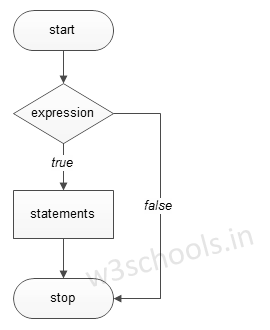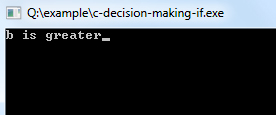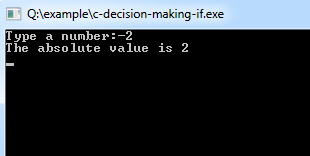## C Programming Tutorial Index

In C, "if statements" control the program flow based on a condition; it executes some statement code block when the expression evaluates to `true`; otherwise, it will get skipped. It is the simplest way to modify the control flow of the program.

The if Statement in C can be used in various forms depending on the situation and complexity.

There are four different types of if statements in C. These are:
• Simple if Statement
• if-else Statement
• Nested if-else Statement

The basic format of the if Statement is:

Syntax:

``````if(test_expression)
{
statement 1;
statement 2;
...
}
``````

'Statement n' can be a statement or a set of statements, and if the test expression is evaluated to `true`, the statement block will get executed, or it will get skipped.

Figure - Flowchart of if Statement:### Example of a C Program to Demonstrate if Statement

Example:

``````#include<stdio.h>

void main()
{
int a = 15, b = 20;

if (b > a) {
printf("b is greater");
}
}``````

Program Output:Example:

``````#include<stdio.h>

void main()
{
int number;
printf("Type a number:");
scanf("%d", &number);

if (number < 0) { // check whether the number is negative number.
number = -number; // If it is a negative then convert it into positive.
printf("The absolute value is %d\n", number);
}
} ``````

Program Output: